# CBSE Class 12 Chemistry Chemical Kinetics MCQs Set A

## Chemical Kinetics Class 12 Chemistry MCQ

Class 12 Chemistry students should refer to the following multiple-choice questions with answers for Chemical Kinetics in standard 12. These MCQ questions with answers for Grade 12 Chemistry will come in exams and help you to score good marks

### Chemical Kinetics MCQ Questions Class 12 Chemistry with Answers

Question : The rate constant of first order reaction is 3 × 10–6 per second. The initial concentration is 0.10 M. The initial rate is:

(a) 3 × 10–7 mol/litre/sec
(b) 3 × 10–8 mol/litre/sec
(c) 3 × 10–5 mol/litre/sec
(d) 3 × 10–8 mol/litre/sec

Question :  Choose the correct options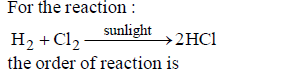(a) 0 (b) 2
(c) 1 (d) 3

Question : Choose the correct options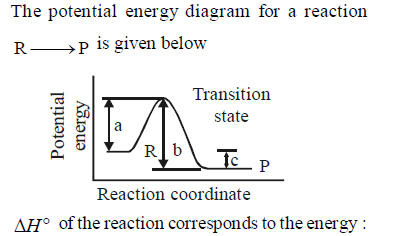(a) a (b) b
(c) c (d) a + b

Question : 4 An endothermic reaction with high activation energy for the forward reaction is given by the diagram :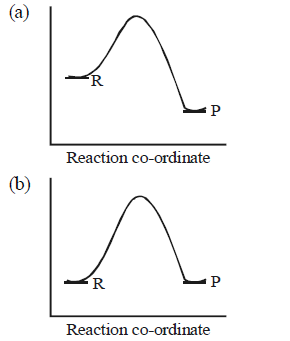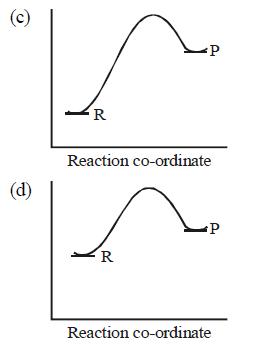Question :  Choose the correct option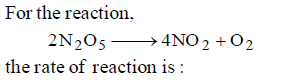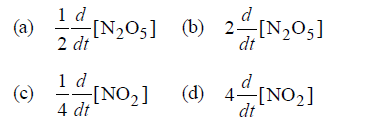Question :  For a first order reaction, to obtain a positive slope, we need to plot {where [A] is the concentration of reactant A}

(a) – log10[A] vs t     (b) – loge[A] vs t
(c) log10[A] vs log t  (d) [A] vs t

Question :  In most cases, for a rise of 10K temperature the rate constant is doubled to tripled. This is due to the reason that

(a) collision frequency increases by a factor of 2 to 3.
(b) fraction of molecules possessing threshold energy increases by a factor of 2 to 3
(c) Activation energy is lowered by a factor of 2 to 3.
(d) none of these

Question :  Half-lives of a first order and a zero order reaction are same. Then the ratio of the initial rates of first order reaction to that of the zero order reaction is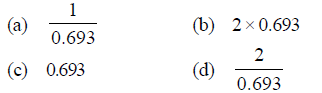Question :  Which of the following relation represents correct relation between standard electrode potential and equilibrium constant?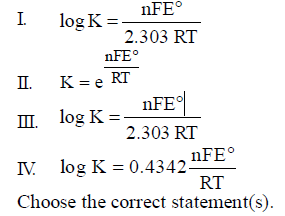(a) I, II and III are correct
(b) II and III are correct
(c) I, II and IV are correct
(d) I and IV are correct

Question : Collision theory is used to explain how chemical species undergo a reaction. Using this theory and the kinetic molecular model, which of the following does NOT influence the rate of a chemical reaction?

(a) The temperature of the system
(b) The geometry or orientation of the collision
(c) The velocity of the reactants at the point ofcollision
(d) All of the above influence the rate

Question : The rate of a chemical reaction tells us about
(a) the reactants taking part in reaction
(b) the products formed in the reaction
(c) how slow or fast the reaction is taking place
(d) none of the above

Question : In the rate equation, when the concentration of reactants is unity then rate is equal to
(a) specific rate constant
(b) average rate constant
(c) stantaneous rate constant
(d)) None of the above

Question : The role of a catalyst is to change
(a) gibbs energy of reaction.
(b) enthalpy of reaction.
(c) activation energy of reaction.
(d) equilibrium constant.

Question : In the presence of a catalyst, the heat evolved or absorbed during the reaction___________.
(a) increases.
(b) decreases.
(c) remains unchanged.
(d) may increase or decrease.

Question : Which of the following statements is not correct about order of a reaction.
(a) The order of a reaction can be a fractional number.
(b) Order of a reaction is experimentally determined quantity.
(c) The order of a reaction is always equal to the sum of the stoichiometriccoefficients of reactants in the balanced chemical equation for a reaction.
(d) The order of a reaction is the sum of the powers of molar concentrationof the reactants in the rate law expression

Question : Which of the following statements is correct?
(a) The rate of a reaction decreases with passage of time as the concentrationof reactants dereases.
(b) The rate of a reaction is same at any time during the reaction.
(c) The rate of a reaction is independent of temperature change.
(d) The  rate  of  a  reaction  decreases  with  increase  in  concentration  ofreactant(s).

Question : Which of the following statements is incorrect about the collison theory of chemical reaction?
(a) It considers reacting molecules or atoms to be hard spheres and ignorestheir structural features.
(b) Number of effective collisions determines the rate of reaction.
(c) Collision of atoms or molecules possessing sufficient threshold energyresults into the product formation.
(d) Molecules should collide with sufficient threshold energy and properorientation for the collision to be effective.

Question : Which of the following statement is not correct for the catalyst?
(a) It catalyses the forward and backward reaction to the same extent.
(b) It alters ∆G of the reaction.
(c) It is a substance that does not change the equilibrium constant of areaction.
(d) It  provides  an  alternate  mechanism  by  reducing  activation  energybetween reactants and products.

Question : The value of rate constant of a pseudo first order reaction ____________.
(a) depends on the concentration of reactants present in small amount.
(b) depends on the concentration of reactants present in excess.
(c) is independent of the concentration of reactants.
(d) depends only on temperature.

Question : The term dc/dt in a rate equation refers to :

(a) the conc. of a reactant

(b) the decrease in conc. of the reactant with time

(c) the velocity constant of reaction

(d) None of these

Question : The rate law for the single- step reaction 2A + B → 2C, is given by:

(a) rate = k [A].[B] (b) rate = k [A]2.[B]

(c) rate = k [2A].[B] (d) rate = k [A]2.[B]o

Question : Rate of which reaction increases with temperature :

(a) of any type of reactions

(b) of exothemic reactions

(c) of endothemic reactions

(d) of none

Question : In a slow reaction, rate of reaction generally .......... with time:

(a) decreases

(b) increases

(c) sometimes increases and sometimes decreases.

(d) remains constant

Question : The rate of a chemical reaction tells us about,

(a) the reactants taking part in reaction

(b) the products formed in the reaction

(c) how slow or fast the reaction is taking place

(d) None of the above

Question :  Point out the wrong statement: For a first order reaction

(a) time for half-change (t1/2) is independent of initial concentration

(b) change in the concentration unit does not change the rate constant (k)

(c) time for half-change × rate constant = 0.693

(d) the unit of k is molemin1

Question :  Consider the reaction, 2A + B → products. When concentration of B alone was doubled, the alf-life did notchange. When the concentration of A alone was doubled, the rate increased by two imes. The unit of rate constant for this reaction is

(a) s–1

(b) L mols1

(c) no unit

(d) mol L–1 s–1.

Question :  The decomposition of N2Ooccurs as 2N2O5 → 4NO+ Oand follows Ist order kinetics, hence:

(a) the reaction is unimolecular

(b) the reaction is bimolecular

(c) t1/2 ∞ a

(d) None of these

Question : Which of the following reaction does not occur fastly ?

(a) Precipitation of AgCl by mixing aqueous solutions of AgNO3 and NaCl.

(b) Burning of gasoline

(c) Rusting of iron

(d) Burning of LPG for cooking

Question : Chemical kinetics is a study to find out

(a) the feasibility of a chemical reaction

(b) extent to which a reaction will proceed

(c) speed of a reaction

(d) All of the above

Question : Rate of a reaction can be defined as

(a) the rate of decrease in concentration of any one of the reactants

(b) the rate of increase in concentration of any one of the products

(c) the rate of decrease in concentration of any one of the reactants or the rate of increase in concentration of any one of the products

(d) the sum of rate of decrease in concentration of all the reactants or the rate of increase in concentration of all the products

Question : The rate of reaction

(a) increases as the reaction proceeds

(b) decreases as the reaction proceeds

(c) remains the same as the reaction proceeds

(d) may decrease or increase as the reaction proceeds

Question : The unit of rate of reaction is

(a) mole/dm3

(b) mole/pound

(c) mole/dm3 sec

(d) mole/cm3

Question : In the rate equation, when the conc. of reactants is unity then rate is equal to

(a) specific rate constant

(b) average rate constant

(c) instantaneous rate constant

(d) None of above

Question : The rate of reaction between two specific time intervals is called

(a) instantaneous rate

(b) average rate

(c) specific rate

(d) ordinary rate

Question : Instantaneous rate of a chemical reaction is

(a) rate of reaction in the beginning

(b) rate of reaction at the end

(c) rate of reaction at a given instant

(d) rate of reaction between two specific time intervals

Question : At the beginning the decrease in the conc. of reactants is

(a) slow

(b) moderate

(c) rapid

(d) None of above

Question : The average rate and instantaneous rate of a reaction are equal

(a) at the start

(b) at the end

(c) in the middle

(d) when two rate have time interval equal to zero

Question : The rate of reaction depends upon the

(a) volume

(b) force

(c) pressure

(d) conc. of reactants

Question : For the following reaction: NO2(g) + CO(g) →NO(g) + CO2(g), the rate law is: Rate = k [NO2]2. If 0.1 mole of gaseous carbon monoxide is added at constant temperatureto the reaction mixture which of the following statements is true?

(a) Both k and the reaction rate remain the same

(b) Both k and the reaction rate increase

(c) Both k and the reaction rate decrease

(d) Only k increases, the reaction rate remain the same

Question : Which one of the following statements for the order of a reaction is incorrect ?

(a) Order can be determined only experimentally.

(b) Order is not influenced by stoichiometric coefficient of the reactants.

(c) Order of reaction is sum of power to the concentration terms of reactants to express the rate of reaction.

(d) Order of reaction is always whole number.

Question : The rate of the reaction 2NO + Cl22NOCl is given by the rate equation rate = k [NO]2 [Cl2]

The value of the rate constant can be increased by:

(a) increasing the concentration of NO.

(b) increasing the temperature.

(c) increasing the concentration of the Cl2

(d) doing all of the above

Question : Order of reaction can be

(a) 0

(b) fraction

(c) whole number

(d) integer, fraction, zero

Question : Units of rate constant of first and zero order reactions in terms of molarity M unit are respectively

(a) sec1, Msec1

(b) sec–1, M

(c) Msec–1, sec–1

(d) M, sec–1.

Question : A reaction involving two different reactants can never be

(a) bimolecular reaction

(b) second order reaction

(c) first order reaction

(d) unimolecular reaction

Question : 3A→B+ C, it would be a zero order reaction when

(a) the rate of reaction is proportional to square of concentration of A

(b) the rate of reaction remains same at any concentration of A

(c) the rate remains unchanged at any concentration of B and C

(d) the rate of reaction doubles if concentration of B is increased to double

Question :  A reaction proceeds by first order, 75% of this reaction was completed in 32 min. The time equired for 50% completion is

(a) 8 min (b) 16 min

(c) 20 min (d) 24 min

Question : Order of reaction is decided by

(a) temperature

(b) mechanism of reaction as well as relative concentration of reactants

(c) molecularity

(d) pressure

Question : Velocity constant k of a reaction is affected by

(a) change in the concentration of the reactant

(b) change of temperature

(c) change in the concentration of the product

(d) None of the above

Question : The rate constant for the reaction

2N2O5 → 4NO2 + O2 is 3.10 × 10–5 sec–1. If the rate is 2.4 × 10–5 mol litre–1 sec–1 then the concentration of N2O(in mol litre–1) is :

(a) 0.04     (b) 0.8

(c) 0.07     (d) 1.4

Question : A zero order reaction is one whose rate is independent of

(a) the concentration of the reactants

(b) the temperature of reaction

(c) the concentration of the product

(d) the material of the vessel in which reaction is carried out

Question : The rate law for a reaction between the substances A and B is given by Rate = k [A]n [B]m On doubling the concentration of A and halving the concentration of B, the ratio of the new rate to the earlier rate of the reaction will be as

(a) (m + n)

(b) (n – m)

(c) 2(n m)

(d) (m n)

Question : In the reaction 2A + B→A2B, if the concentration of A is doubled and that of B is halved, then the rate of the reaction will:

(a) increase 2 times

(b) increase 4 times

(c) decrease 2 times

(d) remain the same

Question : The order of a reaction, with respect to one of the reacting component Y, is zero. It implies that:

(a) the reaction is going on at a constant rate

(b) the rate of reaction does not vary with temperature

(c) the reaction rate is independent of the concentration of Y

(d) the rate of formation of the activated complex is zero

Question : If the rate of a gaseous reaction is independent of pressure, the order of reaction is:

(a) 0

(b) 1

(c) 2

(d) 3

Question : If the rate of the reaction is equal to the rate constant, the order of the reaction is

(a) 3

(b) 0

(c) 1

(d) 2

Question : In a reaction, when the concentration of reactant is increased two times, the increase in rate of reaction was four times. Order of reaction is

(a) zero

(b) 1

(c) 2

(d) 3

Question : For the reaction A + 2B → C, rate is given by R = [A] [B]2 then the order of the reaction is

(a) 3

(b) 6

(c) 5

(d) 7

Question : The unit of rate constant for a zero order reaction is

(a) mol L1 s1

(b) L mol–1 s–1

(c) L2 mol–2 s–1

(d) s–1

Question : Which one of the following reactions is a true first order reaction?

(a) Alkaline hydrolysis of ethyl acetate

(b) Acid catalyst hydrolysis of ethyl acetate

(c) Decomposition of N2O

(d) Decomposition of gaseous ammonia on a hot platinum surface

Question :  Which of the following is not a first order reaction ?

(a) Hydrogenation of ethene

(b) Natural radioactive decay of unstable nuclei

(c) Decomposition of HI on gold surface

(d) Decomposition of N2O

Question : The rate law for the reaction xA + yB → mP + nQ is Rate = k [A]c[B]d. What is the total order of the reaction?

(a) (x + y)

(b) (m + n)

(c) (c + d)

(d) x/y

Question :  Half life of a first order reaction is 4s and the initial concentration of the reactant is 0.12 M. The concentration of the reactant left after 16 s is

(a) 0.0075 M

(b) 0.06 M

(c) 0.03 M

(d) 0.015 M

Question :  The reaction A→ B follows first order kinetics. The timtaken for 0.8 mole of A to produce 0.6 mole of B is 1 hour. What is the time taken for conversion of 0.9 mole of A to produce 0.675 mole of B?

(a) 2 hours

(b) 1 hour

(c) 0.5 hour

(d) 0.25 hour

Question :  The rate of a first order reaction is 1.5 × 10mol Lminat 0.5 M concentration of the reactant. The half life of the reaction is

(a) 0.383 min

(b) 23.1 min

(c) 8.73 min

(d) 7.53 min

Question :  The rate constant for a first order reaction whose half-life, is 480 seconds is :

(a) 2.88 × 10–3 sec–1

(b) 2.72 × 10–3 sec–1

(c) 1.44 × 10 sec1

(d) 1.44 sec–1

Question : The rate constant of a reaction is 3.00 × 103 L mol1 sec1. The order of this reaction will be:

(a) 0

(b) 1

(c) 2

(d) 3

Question :  The rate constant of a first order reaction is 6.9x10-3s-1 . How much time will it take to reduce the initial concentration to its 1/8th value?

(a) 100 s (b) 200 s

(c) 300 s (d) 400 s

Question : For the reaction H2(g) + Br2 (g) → 2HBr (g), the experimental data suggest, rate = k[H2][Br2]1/2. The molecularity and order of the reaction are respectively

(a) 2,   3/2

(b) 3/2 ,3/2

(c) 1, 1

(d) 1, 1/2

Question : Nitrogen monoxide, NO, reacts with hydrogen, H2, according to the following equation:

2NO(g) + 2H2(g) → N2(g) + 2H2O(g)

If the mechanism for this reaction were,

2NO(g) + H2(g) → N2(g) + H2O2(g) ; slow

H2O2(g) + H2(g) → 2H2O(g) ; fast

Which of the following rate laws would we expect to obtain experimentally?

(a) Rate = k[H2O2][H2]

(b) Rate = k[NO]2[H2]

(c) Rate = k[NO]2[H2]2

(d) Rate = k[NO][H2]

## Tags:

Click for more Chemistry Study Material
 CBSE Class 12 Chemistry The Solid State MCQs
 CBSE Class 12 Chemistry Electrochemistry MCQs
 CBSE Class 12 Chemistry Surface Chemistry MCQs
 CBSE Class 12 Chemistry General Principles and Processes of Isolation of Elements MCQs
 CBSE Class 12 Chemistry The p Block Elements MCQs
 CBSE Class 12 Chemistry The d and f Block Elements MCQs
 CBSE Class 12 Chemistry Coordination Compounds MCQs
 CBSE Class 12 Chemistry Haloalkanes and Haloarenes MCQs
 CBSE Class 12 Chemistry Alcohols Phenols and Ethers MCQs
 CBSE Class 12 Chemistry Aldehydes Ketones and Carboxylic Acids MCQs
 CBSE Class 12 Chemistry Amines MCQs
 CBSE Class 12 Chemistry Biomolecules MCQs
 CBSE Class 12 Chemistry Polymers MCQs
 CBSE Class 12 Chemistry Chemistry in Everyday Life MCQs

## Latest NCERT & CBSE News

Read the latest news and announcements from NCERT and CBSE below. Important updates relating to your studies which will help you to keep yourself updated with latest happenings in school level education. Keep yourself updated with all latest news and also read articles from teachers which will help you to improve your studies, increase motivation level and promote faster learning

### Heritage India Quiz 2021 2022

CBSE Heritage India Quiz is conducted every year to raise the awareness about the preserving human heritage, diversity and vulnerability of the India's built monuments and heritage sites. It is an attempt of the Board to motivate the future generations of this country...

### Board Exams Date Sheet Class 10 and Class 12

Datesheet for CBSE Board Exams Class 10  (Scroll down for Class 12 Datesheet) Datesheet for CBSE Board Exams Class 12

### CBSE Term 2 Board Examinations

CBSE vide Circular No.Acad-51/2021 dated 5th July, 2021, notified that in the session 2021-2022, Board Examinations would be conducted in two terms, i.e.. Term I and Term II. This decision was taken due to the uncertainty arising out of COVID 19 Pandemic. Term I...

### All India Children Educational Audio Video Festival

The Central Institute of Educational Technology (CIET), a constituent unit of National Council of Educational Research and Training (NCERT), is inviting entries for the 26th All India Children’s Educational Audio Video Festival (AICEAVF). This festival showcases the...